## Wednesday, November 30, 2011

### Air Fundamentals

Pressure can be measured in absolute terms or gauge. The only difference is the reference point. When we refer to absolute pressure we refer to the pressure with respect to absolute zero i.e. total vacuum. While referring to gauge pressure, atmosphere is taken as datum - zero and pressure above atmosphere is termed as gauge pressure. For example if we measure height of mountain with respect to the mean sea level then it is the absolute height of mountain from mean sea level. However, if we measure height of mountain from its foot we talk of relative height of the mountain with respect to its foot and in order to get the absolute height of the mountain w.r.t. mean sea level we must add the height of mountain foot from mean sea level. For most of pressure applications, gauge pressures are generally referred to. However, sometimes people use absolute pressures in which case suffix “abs” is indicated.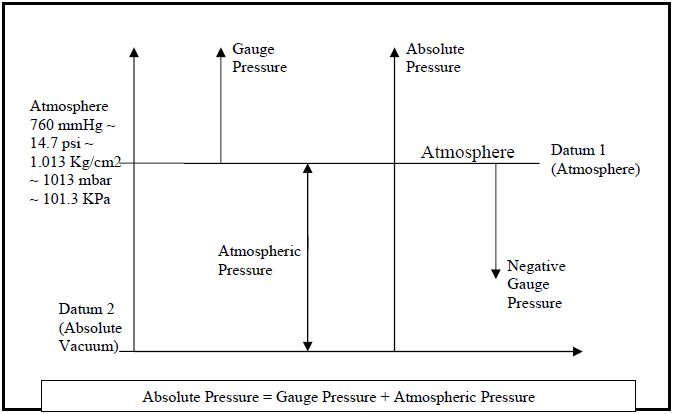Examples:
1. 1 psig means 1 psi above atmospheric pressure. To convert to absolute units we add 14.7 psi (Atmospheric Pressure) to 1 psi, to get 15.7 psi(A). Therefore we have 1 psig = 15.7 psi(A).

2. Similarly, 6 psig = 14.7 + 6 psi(A) = 20.7 psi(A)

3. For vacuum, we refer to pressure below the atmospheric level. Vacuum can be designated both in psig or psi(A). For Example -5 psi vacuum indicates that you are below the atmosphere by 5 psi. Taking datum 1 (atmosphere) we would refer as -5 psig, in absolute units it would be 14.7-5=9.7 psi(A).

Air is a compressible fluid which expands to the available volume. For this reason whenever volume is specified, pressure and temperature have to be indicated. As per Charles Gas Law P1V1/T1 = P2V2/T2, for all gases. Internationally, accepted standard conditions for temperature and pressure are specified, which are denoted by NTP (Normal Temperature & Pressure) and STP (Standard Temperature & Pressure). For NTP conditions, pressure is taken as 1 Atmosphere (~ 14.7 psi(A) ~ 1013 mbar) and temperature is 0°C (~273 K). For STP conditions, reference pressure is 1 Atmosphere (~14.7 psi(A) ~ 1013 mbar) and temperature is 20°C (~293 K).

Whenever pressure and temperature are used in a gas equation they have to be converted to absolute units of temperature and pressure. When a client specifies volume, he generally refers to SCFM & when he specifies volume in terms of NCFM or Nm3/hr, he is refers to volume at NTP conditions.

Roots blowers are designed for suction capacity which is referred to as ICFM (Inlet Cubic Feet per Minute) or ACFM (Actual Cubic Feet per Minute) or FAD (Free Air Delivery at Inlet Conditions) . Our catalogue gives the values as CFM which is actually ICFM or ACFM. In many cases clients indicate capacity in Nm3/hr or NCFM which is to be converted by the gas law to find out equivalent capacity in ICFM or ACFM to size the blower. Few examples are illustrated below:

1. Client needs 100 Nm3/hr at 6 psig discharge pressure.
We need to select blower having inlet capacity of 100 Nm3/hr and capable of
handling discharge back pressure of 6 psi. We, therefore, convert the Nm3/hr
capacity, to blower inlet capacity.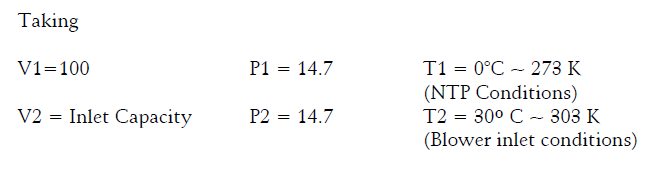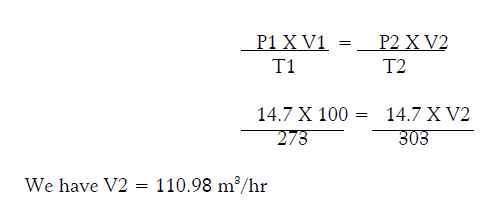Blower selection may be done accordingly from the Blower selection chart, for the capacity 111 m3/hr at 6 psig differential pressure.

2. Customer wants 100 SCFM Air Blower at 6 psig for installation at an elevation where atmospheric pressure is 13.5 psi(A).
For this case since client has indicated inlet capacity in SCFM conditions i.e. Pressure 14.7 psi and temperature 20°C, whereas he has to run the blower at an elevation where atmospheric pressure is 13.7 psi(A) which indicates that blower inlet capacity at that pressure should be more. Therefore in order to calculate inlet capacity, at elevated area of operation, we use the gas law,
where,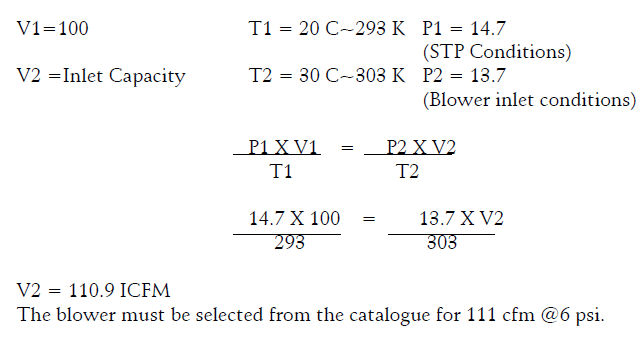3. Let us consider 1000 SCFM ; 7 psi(A) Inlet & 14.4 psi(A) outlet
For this case the capacity is given in SCFM where P1 = 14.7 and T1 = 20º C. The blower is to run at 7 psi(A) which is 7 psi Absolute Pressure or (14.4 –7)= – 7.4 psig. Taking 14.4 as atmospheric pressure at the site, the suction pressure at blower inlet would be 7 psi(A) and the differential pressure across the blower as 7.4 psi. The capacity 1000 SCFM must be converted to volume at blower inlet condition i.e. P2=7 psi(A) and T2=Say 30º C.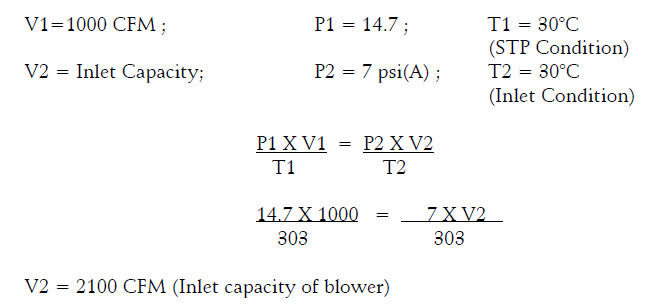The blower must be sized for Inlet Capacity 2100 CFM and differential pressure 7.4 psi from the blower selection chart.
4. Clients needs a blower for 200 cfm, 3 psi.
In this case since nothing is specified it may be taken that the blower specification is to be worked out taking the above as inlet capacity. This is generally the condition encountered in most of the cases.

5. In rare case, client may indicate requirement of air blower for compressed volume of 100 cfm at discharge pressure of 5 psig.
In this case he is referring to the discharge compressed volume of 100 cfm at discharge pressure of 5 psig. We must calculate the free air inlet volume (FAD) at inlet conditions, to size the blower.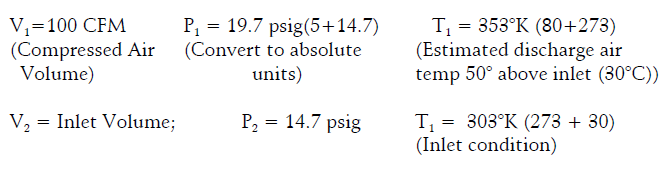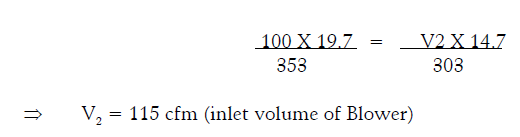6. In certain cases clients may indicate mass flow rate in kg/hr for which using the formula PV = ρRT where P1, V1 & T1 are pressure, volumetric flow rate & temperature at inlet conditions, ρ is density & R is Universal Gas Constant we can calculate the air volume at specified conditions.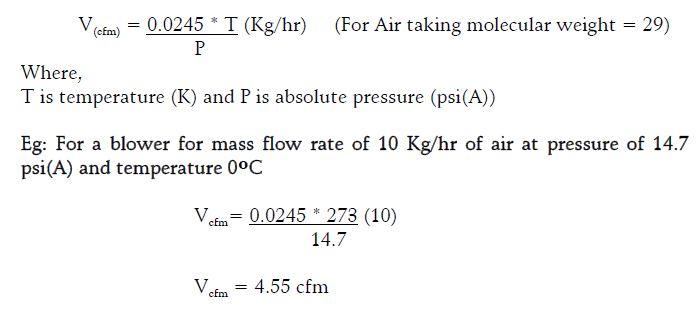One must clearly understand that air being compressible fluid, conditions of temperature and pressure must match to that on which blower selection chart has been prepared so that selection can be made. It is same as if item is priced in US \$, the same must be multiplied by a constant to get equivalent price in any other currency.